# Seaborn Line Plot using sns.lineplot() – Tutorial for Beginners with Example## Introduction

In this article, we will go through the Seaborn line plot tutorial for your machine learning or data science projects. We will understand the syntax of the lineplot() function of the Seaborn library and see various examples for easy understanding of beginners. Let’s start the tutorial now.

## Seaborn Line Plot Tutorial

Line plot is a very common visualization that helps to visualize the relationship between two variables by drawing the line across the data points.

There is a function lineplot() in Seaborn library that can be used to easily generate beautiful line plots. In the next section, we will see the syntax of lineplot() along with its different parameters

### Syntax

seaborn.lineplot(*, x=None, y=None, hue=None, size=None, style=None, data=None, palette=None, hue_order=None, hue_norm=None, sizes=None, size_order=None, size_norm=None, dashes=True, markers=None, style_order=None, units=None, estimator=’mean’, ci=95, n_boot=1000, seed=None, sort=True, err_style=’band’, err_kws=None, legend=’auto’, ax=None, kwargs)**

x, y : vectors or keys in data
This parameter helps in specifying

hue : vector or key in data
The grouping based on hue will produce lines of different colors.

size : vector or key in data
The size parameter helps in producing lines of different sizes.

style : vector or key in data
This parameter can change the style of lines.

data : pandas.DataFrame, numpy.ndarray, mapping, or sequence
Here we provide the data for the visualization.

palette : string, list, dict, or matplotlib.colors.Colormap
This method helps in selecting colors for the plot.

sizes : list, dict, or tuple
This parameter sets the sizes for the size.

size_norm : tuple or Normalize object
Normalization in data units for scaling plot objects when the size variable is numeric.

dashes : boolean, list, or dictionary
This object determines the way in which lines will be drawn for several levels of style variable

markers : boolean, list, or dictionary
Here we determine the markers for different levels.

legend : “auto”, “brief”, “full”, or False
Here we specify the way in which the legend is displayed on the visualization.

ax : matplotlib.axes.Axes
Pre-existing axes for the plot. Otherwise, call matplotlib.pyplot.gca() internally.

## Seaborn Line Plot Example

Let us now have a look at some examples of lineplot() in seaborn.

### 1st Example – Line Plot in Seaborn using Long-Form Data

In this example, we will use long-form data for creating a line plot in seaborn. Long-form data is the format in which variables of the dataset are represented by column and each observation is denoted by a row.

Here we will first load seaborn’s in-built dataset “flights” and then we fetch only April month data for our purpose.

After this, we call lineplot() function for plotting the line plot. We pass the x and y parameter as “years” and “passenger” respectively.

In :
```import seaborn as sns

```

Output:

year month passengers
0 1949 Jan 112
1 1949 Feb 118
2 1949 Mar 132
3 1949 Apr 129
4 1949 May 121
In :
```apr_flights = flights.query("month == 'Apr'")
sns.lineplot(data=apr_flights, x="year", y="passengers")
```
Output: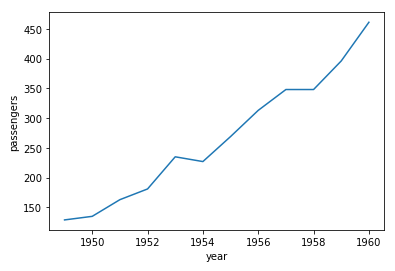### 2nd Example – Line Plot in Seaborn using Wide-Form Data

In this second example, we will create a seaborn line plot using wide-form data. In wide-form format, data is laid down in a manner where both columns and rows consist of variables like a matrix form.

For converting our dataset into wide-form we will apply pivot and then use lineplot() to create the line plot.

In :
```flights_wide = flights.pivot("year", "month", "passengers")
```
Output:
month Jan Feb Mar Apr May Jun Jul Aug Sep Oct Nov Dec
year
1949 112 118 132 129 121 135 148 148 136 119 104 118
1950 115 126 141 135 125 149 170 170 158 133 114 140
1951 145 150 178 163 172 178 199 199 184 162 146 166
1952 171 180 193 181 183 218 230 242 209 191 172 194
1953 196 196 236 235 229 243 264 272 237 211 180 201
In :
```sns.lineplot(data=flights_wide["Jul"])
```
Output: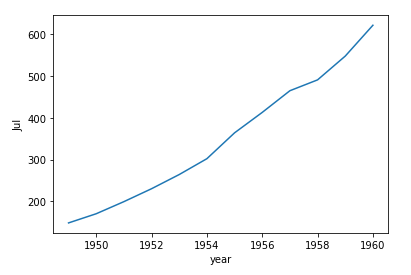### 3rd Example – Passing entire long-form data and categorizing with Hue

In this example, we will pass the entire data to lineplot() function and use the hue parameter for categorization with month.

We also use the rainbow color palette to make categories more distinctively visible.

In :
```sns.lineplot(data=flights, x="year", y="passengers", hue="month", palette="rainbow")
```
Output: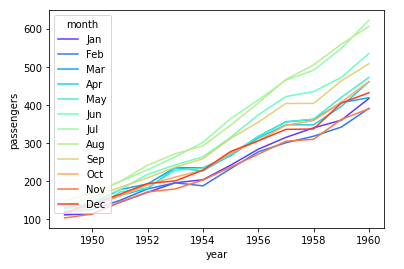### 4th Example – Aggregation of Repeating Observations

In this current example, we are going to use another dataset of seaborn. Once we load that dataset, we pass data, x, y, and hue.

This dataset is used here because we want to visualize repeating observations.

In :
```fmri = sns.load_dataset("fmri")
```
Output:
subject timepoint event region signal
0 s13 18 stim parietal -0.017552
1 s5 14 stim parietal -0.080883
2 s12 18 stim parietal -0.081033
3 s11 18 stim parietal -0.046134
4 s10 18 stim parietal -0.037970
In :
```sns.lineplot(data=fmri, x="timepoint", y="signal", hue="event")
```
Output: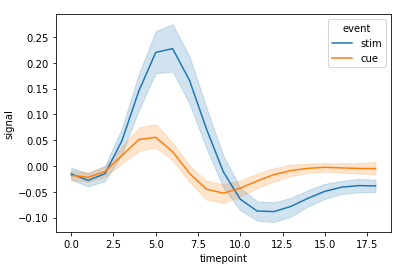### 5th Example – Using Hue and Style to represent two different grouping variables

For this example, we can perform grouping based on multiple variables. To achieve this, we use both hue and style parameters.

According to the dataset, we get multiple variables, these can be visualized using the below method.

In :
```sns.lineplot(data=fmri, x="timepoint", y="signal", hue="region", style="event")
```
Output: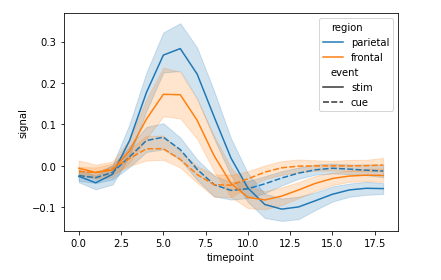### 6th Example – Using a numeric variable for grouping with Hue

In this example, we’ll look at the way of grouping numeric variables.

We will import dataset for this purpose and then use the query function to access desired data.

At last, we call lineplot() function for creating the plot

In :
```dots = sns.load_dataset("dots").query("align == 'dots'")
```
Output:
align choice time coherence firing_rate
0 dots T1 -80 0.0 33.189967
1 dots T1 -80 3.2 31.691726
2 dots T1 -80 6.4 34.279840
3 dots T1 -80 12.8 32.631874
4 dots T1 -80 25.6 35.060487
In :
```sns.lineplot(
data=dots, x="time", y="firing_rate", hue="coherence", style="choice",palette="Greens"
)
```
Output: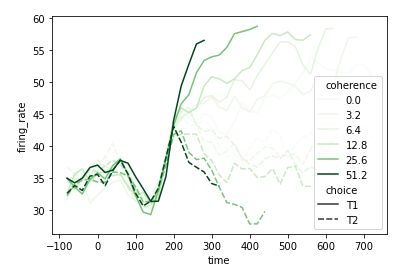## Conclusion

We have reached the end of this seaborn tutorial for line plot where we learned lineplot() function and its syntax. We also saw different examples of creating line plots by making use of various parameters and datasets.

Reference: https://seaborn.pydata.org/